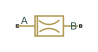# Fixed Orifice Empirical

(To be removed) Fixed hydraulic orifice based on empirical data

The Hydraulics (Isothermal) library will be removed in a future release. Use the Isothermal Liquid library instead. (since R2020a)

## Library

Orifices

•## Description

The Fixed Orifice Empirical block models a fixed orifice in hydraulic systems with data obtained from experimental testing. The data required for characterization is the set of pressure differentials across the orifice, together with associated flow rates determined for a particular fluid and temperature.

The flow rate through the orifice is determined with the table lookup block from the set of experimental measurements

q = `f`(p)

where

 `q` Volumetric flow rate `p` Pressure differential

You can select from two interpolation and two extrapolation methods.

Note

Extrapolation could result in large errors if the operating point deviates significantly from the area of experiments.

The block positive direction is from port A to port B. This means that the flow rate is positive if it flows from A to B, and the pressure differential is determined as $\Delta p={p}_{\text{A}}-{p}_{\text{B}},$.

## Parameters

Tabulated pressure differentials

Specify the pressure differential vector as a one-dimensional array. The vector contains values of pressure differentials obtained during experimental characterization of the orifice. The vector must be strictly increasing. The values can be nonuniformly spaced. The minimum number of values depends on the interpolation method: you must provide at least two values for linear interpolation, at least three values for smooth interpolation. The default values, in bar, are ```[-40.0, -30.0, -20.0, -10.0, -5.0, 0.0, 5.0, 10.0, 20.0, 30.0, 40.0]```.

Tabulated flow rates

Specify the vector of flow rates through the orifice, corresponding to these pressure differentials, as a one-dimensional array. The vector must be of the same size as the Tabulated pressure differentials vector. The default values, in m^3/s, are ```[ -0.0024436 -0.0021248 -0.0016852 -0.0012248 -8.4476e-4 0 8.5333e-4 0.0012068 0.0017067 0.0020902 0.0024136 ]```.

Interpolation method

Select one of the following interpolation methods for approximating the output value when the input value is between two consecutive grid points:

• `Linear` — Select this option to get the best performance.

• `Smooth` — Select this option to produce a continuous curve with continuous first-order derivatives.

For more information on interpolation algorithms, see the PS Lookup Table (1D) block reference page.

Extrapolation method

Use extrapolation with extreme caution, because moving beyond the measurement limits could result in large errors. Select one of the following extrapolation methods for determining the output value when the input value is outside the range specified in the argument list:

• `Linear` — Select this option to produce a curve with continuous first-order derivatives in the extrapolation region and at the boundary with the interpolation region.

• `Nearest` — Select this option to produce an extrapolation that does not go above the highest point in the data or below the lowest point in the data.

For more information on extrapolation algorithms, see the PS Lookup Table (1D) block reference page.

## Ports

The block has the following ports:

`A`

Hydraulic conserving port associated with the orifice inlet.

`B`

Hydraulic conserving port associated with the orifice outlet.

## Version History

Introduced in R2012b

expand all